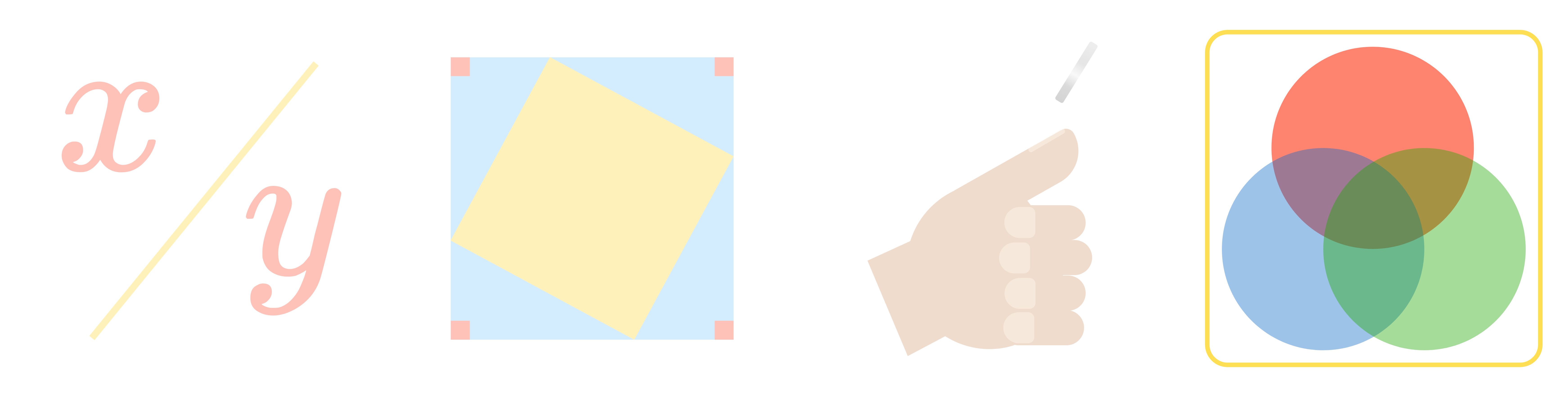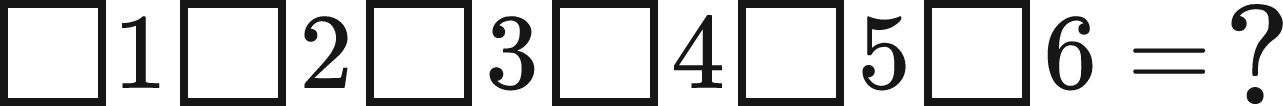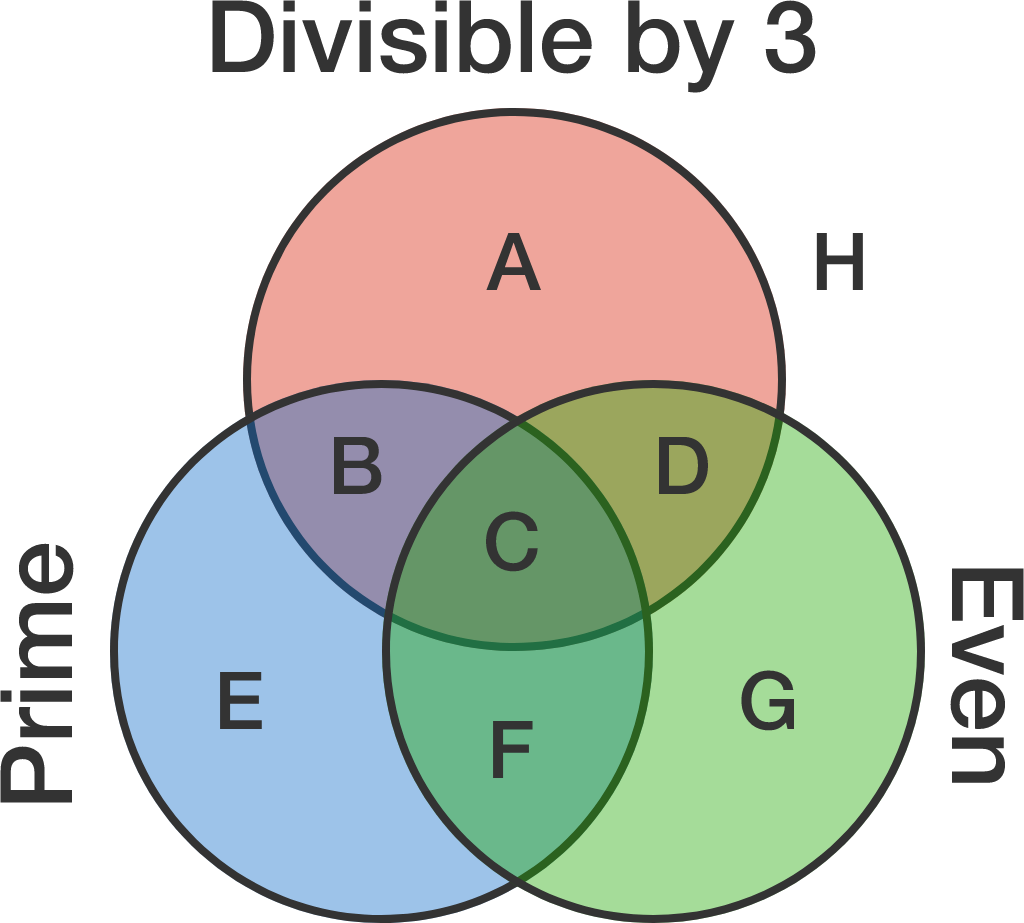Contest Math INumber theorists study the relationships between numbers, particularly the integers. Number theory in math competitions delves into the less known and often surprising relationships that exist between numbers. To solve problems like the ones in this quiz, you will need to understand relationships like those between prime and composite numbers, the rules for determining divisibility by a digit, or the connections between odd and even numbers.

Number Theory

Let $n$ be a two-digit number that is divisible by 9. When the digits of $n$ are reversed, the number formed is divisible by 5. What is the value of $n?$

Number Theory

I'm thinking of two positive whole numbers that multiply to 100, neither of which contains the digit 0. What is the sum of these 2 numbers?Number Theory

If you fill each of the boxes below with either a "+" or a "-" sign, will the resulting sum be odd or even?Number TheoryIf this Venn diagram is filled with all positive integers, which of the regions marked with a letter will be empty?

Number Theory

Select one or more

If $a = 1 + 2 + 3 + 4 + 5 + 6 + 7 + 8 + 9 + 10$ and $b = 1 \times 2 \times 3 \times 4 \times 5 \times 6\ \times 7 \times 8 \times 9 \times 10,$ what is the greatest common factor of $a$ and $b?$

Number Theory

The number theory questions in math competitions frequently focus on efficiency or numerical relationships. We have units on each of these categories in this course, and you don't need to work through all of the units in this course in order, you can jump directly to whichever one you want to practice.

The unit on Efficiency will provide you with a wide array of tools that will save you a lot of time and effort in math competitions, by shortening calculations or even skipping them entirely. It will also show you how to use relationships between numbers to work backwards and find unknowns. To learn more, you can either explore what's in this unit or read through the Number Theory: Efficiency Syllabus.

The unit on Factorization explores the relationships between numbers based on their factors. You will learn to use the prime factorization to find common divisors and multiples as well as ways to apply divisibility rules efficiently. To learn more, you can either explore what's in this unit or read through the Number Theory: Factorization Syllabus.

×